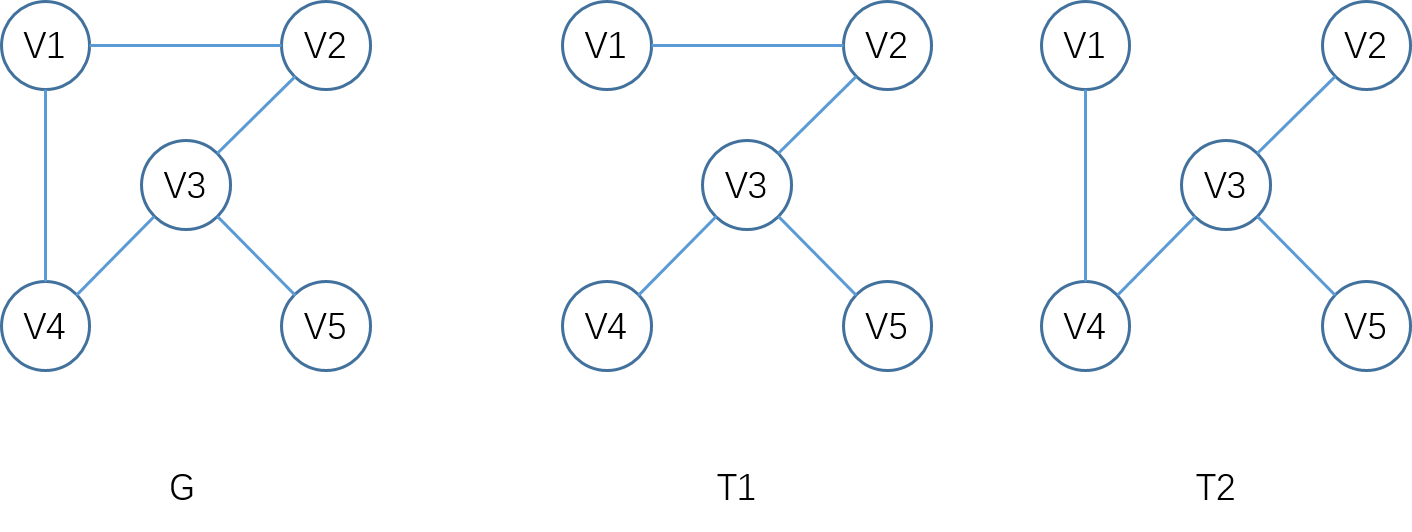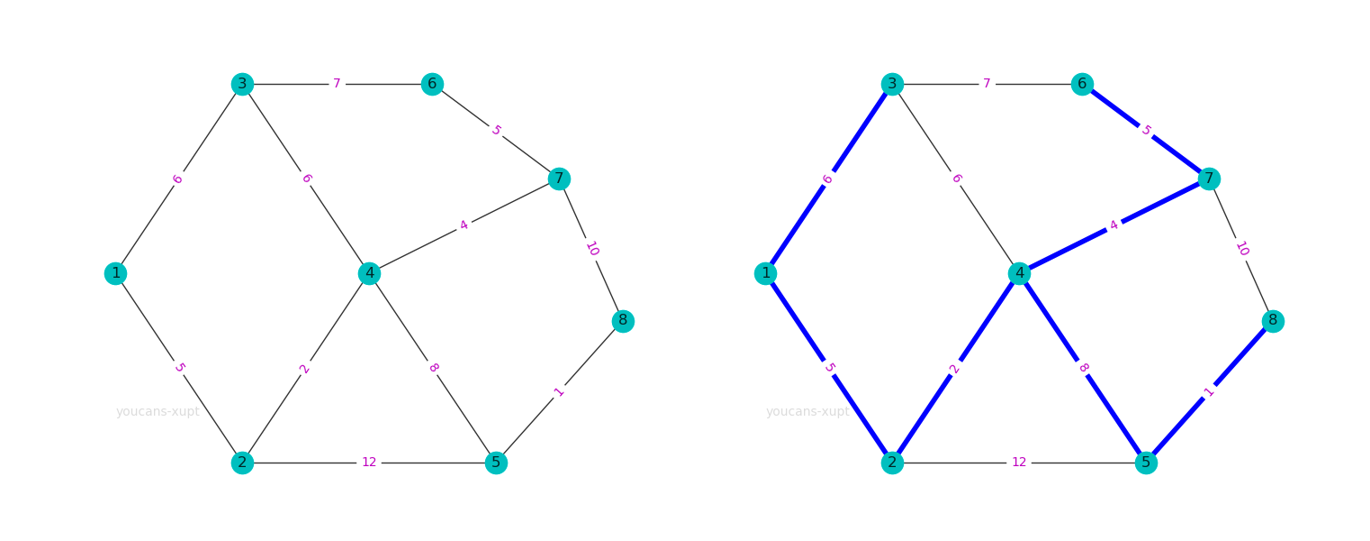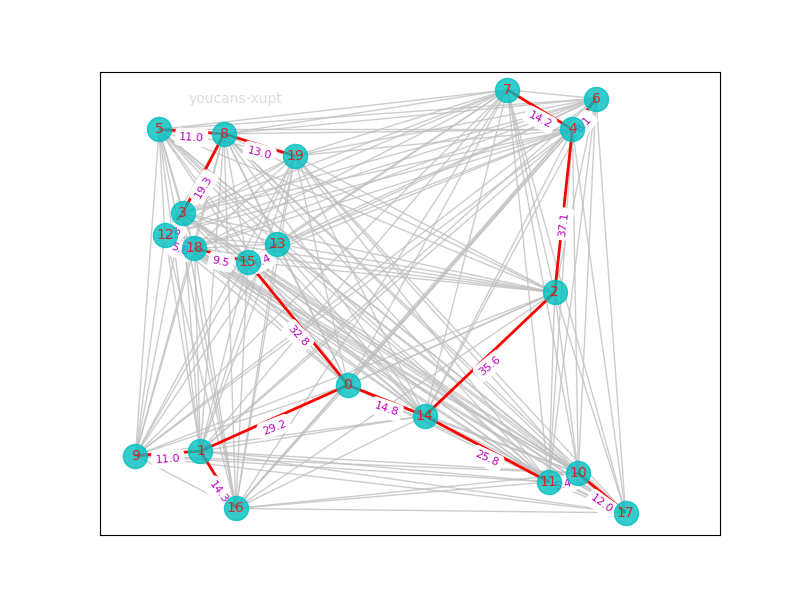# Python小白的数学建模课-18.最小生成树问题

• 最小生成树（MST）是图论中的基本问题，具有广泛的实际应用，在数学建模中也经常出现。
• 路线设计、道路规划、官网布局、公交路线、网络设计，都可以转化为最小生成树问题，如要求总线路长度最短、材料最少、成本最低、耗时最小。
• 最小生成树的典型算法有普里姆算法（Prim算法）和克鲁斯卡算法（Kruskal算法）.
• 本文基于 NetworkX 工具包，通过例程详细介绍最小生成树问题的求解。
• 『Python小白的数学建模课 @ Youcans』带你从数模小白成为国赛达人。

## 1. 最小生成树

### 1.1 生成树## 2. 最小生成树算法

### 2.1 普里姆算法（Prim算法）

Prim 算法以顶点为基础构造最小生成树，每个顶点只与连通图连接一次，因此不用考虑在加入顶点的过程中是否会形成回路。

Prim 算法中图的存贮结构采用邻接矩阵，使用一个顶点集合 u 构造最小生成树。由于不断向集合u中加点，还需要建立一个辅助数组来同步更新最小代价边的信息。

Prim 算法每次选择顶点时，都需要进行排序，但每次都只需要对一部分边进行排序。Prim 算法的时间复杂度为 O(n*n)，与边的数量无关，适用于边很多的稠密图。

### 2.2 克鲁斯卡算法（Kruskal算法）

Kruskal 算法以边为基础构造最小生成树，利用避圈思想，每次找到不使图构成回路的代价最小的边。

Kruskal 算法中图的存贮结构采用边集数组，权值相等的边在数组中的排列次序是任意的。Kruskal算法开始就要对所有的边进行排序，之后还需要对所有边应用 Union-Find算法，但不再需要排序。

Kruskal 算法的时间复杂度为 O(mlogm)，主要是对边排序的时间复杂度，适用于边较少的稀疏图。

## 3. NetworkX 的最小生成树算法

### 3.1 NetworkX 的最小/最大生成树函数

minimum_spanning_tree(G[, weight,...]) 计算无向图上的最小生成树
maximum_spanning_tree(G[, weight,...]) 计算无向图上的最大生成树
minimum_spanning_edges(G[, algorithm,...]) 计算无向加权图最小生成树的边
maximum_spanning_edges(G[, algorithm,...]) 计算无向加权图最大生成树的边

### 3.2 minimum_spanning_tree() 使用说明

minimum_spanning_tree(G, weight='weight', algorithm='kruskal', ignore_nan=False)

minimum_spanning_edges(G, algorithm='kruskal', weight='weight', keys=True, data=True, ignore_nan=False)

minimum_spanning_tree() 用于计算无向连通图的最小生成树（森林）。minimum_spanning_edges() 用于计算无向连通图的最小生成树（森林）的边。

• G(undirected graph)：无向图。
• weight(str)：指定用作计算权重的边属性。
• algorithm(string)：计算最小生成树的算法，可选项为 'kruskal'、'prim' 或 'boruvka'。默认算法为 'kruskal'。
• data(bool)：指定返回值是否包括边的权值。
• ignore_nan(bool) ：在边的权重为 Nan 时产生异常。

• minimum_spanning_tree() 的返回值是由最小生成树构成的图，类型为 NetworkX Graph，需要用 T.edges() 获得对应的最小生成树的边。
• minimum_spanning_edges() 的返回值是最小生成树的构成边，类型为<class 'generator'>，需要用 list() 转换为列表数据。

### 3.3 案例：天然气管道铺设问题1. 图的输入。本例为稀疏的有权无向图，使用 G.add_weighted_edges_from() 函数以列表向图中添加多条赋权边，每个赋权边以元组 (node1,node2,weight) 表示。
2. nx.minimum_spanning_tree() 和 nx.tree.minimum_spanning_edges() 都可以计算最小生成树，参数设置和属性也基本一致，区别主要在于返回值的格式和调用方式。

Python 例程：

# mathmodel18_v1.py
# Demo18 of mathematical modeling algorithm
# Demo of minimum spanning tree(MST) with NetworkX
# Crated：2021-07-10

import numpy as np
import matplotlib.pyplot as plt # 导入 Matplotlib 工具包
import networkx as nx  # 导入 NetworkX 工具包

# 1. 天然气管道铺设
G1 = nx.Graph()  # 创建：空的 无向图
(3,6,7),(4,5,8),(4,7,4),(5,8,1),(6,7,5),(7,8,10)])  # 向图中添加多条赋权边: (node1,node2,weight)

T = nx.minimum_spanning_tree(G1)  # 返回包括最小生成树的图
print(T.nodes)  # 最小生成树的 顶点
print(T.edges)  # 最小生成树的 边
print(sorted(T.edges)) # 排序后的 最小生成树的 边
print(sorted(T.edges(data=True)))  # data=True 表示返回值包括边的权重

mst1 = nx.tree.minimum_spanning_edges(G1, algorithm="kruskal") # 返回最小生成树的边
print(list(mst1))  # 最小生成树的 边
mst2 = nx.tree.minimum_spanning_edges(G1, algorithm="prim",data=False)  # data=False 表示返回值不带权
print(list(mst2))

# 绘图
pos={1:(1,5),2:(3,1),3:(3,9),4:(5,5),5:(7,1),6:(6,9),7:(8,7),8:(9,4)}  # 指定顶点位置
nx.draw(G1, pos, with_labels=True, node_color='c', alpha=0.8)  # 绘制无向图
labels = nx.get_edge_attributes(G1,'weight')
nx.draw_networkx_edge_labels(G1,pos,edge_labels=labels, font_color='m') # 显示边的权值
nx.draw_networkx_edges(G1,pos,edgelist=T.edges,edge_color='b',width=4)  # 设置指定边的颜色
plt.show()


[1, 2, 3, 4, 5, 6, 7, 8]
[(1, 2), (1, 3), (2, 4), (4, 7), (4, 5), (5, 8), (6, 7)]
[(1, 2), (1, 3), (2, 4), (4, 5), (4, 7), (5, 8), (6, 7)]
[(1, 2, {'weight': 5}), (1, 3, {'weight': 6}), (2, 4, {'weight': 2}), (4, 5, {'weight': 8}), (4, 7, {'weight': 4}), (5, 8, {'weight': 1}), (6, 7, {'weight': 5})]
[(5, 8, {'weight': 1}), (2, 4, {'weight': 2}), (4, 7, {'weight': 4}), (1, 2, {'weight': 5}), (6, 7, {'weight': 5}), (1, 3, {'weight': 6}), (4, 5, {'weight': 8})]
[(1, 2), (2, 4), (4, 7), (7, 6), (1, 3), (4, 5), (5, 8)]


## 4. 案例：建设通信网络

### 4.1 问题描述

（1）城市数$n\geq10$，由键盘输入；
（2）城市坐标 x, y 在（0～100）之间随机生成；
（3）输出线路方案的各段线路及长度。

### 4.2 程序说明

1. 这是一个典型的最小生成树问题。n 个城市构成图的 n 个顶点，任意两个顶点之间都有连接边，边的权值是两个顶点的间距。
2. nx.complete_graph(n) 可以创建一个全连接图，即任意两个顶点之间都有连接边。

### 4.3 Python 例程

# mathmodel18_v1.py
# Demo18 of mathematical modeling algorithm
# Demo of minimum spanning tree(MST) with NetworkX
# Crated：2021-07-10

import numpy as np
import matplotlib.pyplot as plt # 导入 Matplotlib 工具包
import networkx as nx  # 导入 NetworkX 工具包
from scipy.spatial.distance import pdist, squareform

# # 2. 城市通信网络建设
# nCities = input("Input number of cities (n>=10):")
# nCities = int(nCities)
nCities = 20
np.random.seed(1)
xPos = np.random.randint(0, 100, nCities)  # 生成 [0,100) 均匀分布的随机整数
yPos = np.random.randint(0, 100, nCities)  # 生成 Ncities 个城市坐标

posCity = []
G2 = nx.complete_graph(nCities)  # 创建：全连接图
for node in G2.nodes():
G2.add_node(node, pos=(xPos[node], yPos[node]))  # 向节点添加位置属性 pos
posCity.append(G2.nodes[node]["pos"])  # 获取节点位置属性 pos

dist = squareform(pdist(np.array(posCity)))  # 计算所有节点之间的距离
for u, v in G2.edges:
G2.add_edge(u, v, weight=np.round(dist[u][v],decimals=1))  # 向边添加权值 dist(u,v)

T = nx.minimum_spanning_tree(G2, algorithm='kruskal')  # 返回包括最小生成树的图
print("\n城市位置:\n",G2._node)
print("\n通信网络:\n",sorted(T.edges(data=True)))  # data=True 表示返回值包括边的权重
# mst = nx.tree.minimum_spanning_edges(G2, algorithm="kruskal") # 返回最小生成树的边
# for edge in sorted(list(mst)):
#     print(edge)

fig, ax = plt.subplots(figsize=(8, 6))
node_pos = nx.get_node_attributes(G2, 'pos')  # 顶点位置
nx.draw(G2,node_pos,with_labels=True,node_color='c',edge_color='silver',node_size=300,font_size=10,font_color='r',alpha=0.8)  # 绘制无向图
# nx.draw_networkx_labels(G2, node_pos, labels=node_pos, font_size=6, horizontalalignment='left', verticalalignment='top')  # 绘制顶点属性：位置坐标 pos
# edge_col = ['red' if edge in T.edges() else 'silver' for edge in G2.edges()]  # 设置边的颜色
# nx.draw_networkx_edges(G2, node_pos, edge_color=edge_col, width=2)  # 设置指定边的颜色
nx.draw_networkx_edges(G2, node_pos, edgelist=T.edges, edge_color='r', width=2)  # 设置指定边的颜色
edge_weight = nx.get_edge_attributes(T, 'weight')  # 边的权值
nx.draw_networkx_edge_labels(T, node_pos, edge_labels=edge_weight, font_size=8, font_color='m', verticalalignment='top')  # 显示边的权值
plt.axis('on')  # Remove the axis
plt.xlim(-5, 100)
plt.ylim(-5, 100)
plt.show()


### 4.4 运行结果

城市位置:
{0: {'pos': (37, 29)}, 1: {'pos': (12, 14)}, 2: {'pos': (72, 50)}, 3: {'pos': (9, 68)}, 4: {'pos': (75, 87)}, 5: {'pos': (5, 87)}, 6: {'pos': (79, 94)}, 7: {'pos': (64, 96)}, 8: {'pos': (16, 86)}, 9: {'pos': (1, 13)}, 10: {'pos': (76, 9)}, 11: {'pos': (71, 7)}, 12: {'pos': (6, 63)}, 13: {'pos': (25, 61)}, 14: {'pos': (50, 22)}, 15: {'pos': (20, 57)}, 16: {'pos': (18, 1)}, 17: {'pos': (84, 0)}, 18: {'pos': (11, 60)}, 19: {'pos': (28, 81)}}

[(0, 1, {'weight': 29.2}), (0, 14, {'weight': 14.8}), (0, 15, {'weight': 32.8}), (1, 9, {'weight': 11.0}), (1, 16, {'weight': 14.3}), (2, 4, {'weight': 37.1}), (2, 14, {'weight': 35.6}), (3, 8, {'weight': 19.3}), (3, 12, {'weight': 5.8}), (4, 6, {'weight': 8.1}), (4, 7, {'weight': 14.2}), (5, 8, {'weight': 11.0}), (8, 19, {'weight': 13.0}), (10, 11, {'weight': 5.4}), (10, 17, {'weight': 12.0}), (11, 14, {'weight': 25.8}), (12, 18, {'weight': 5.8}), (13, 15, {'weight': 6.4}), (15, 18, {'weight': 9.5})]【本节完】